## Selina Concise Mathematics Class 10 ICSE Solutions Chapter 25 Probability Ex 25B

These Solutions are part of Selina Concise Mathematics Class 10 ICSE Solutions. Here we have given Chapter 25 Probability Ex 25B.

Other Exercises

Question 1.
Nine cards (identical in all respects) are numbered 2 to 10. A card is selected from them at random. Find the probability that the card selected will be :
(i) an even number.
(ii) a multiple of 3 ,
(iii) an even number and a multiple of 3.
(iv) an even number or a multiple of 3.
Solution:
No. of cards = 9
Having numbers marked on it = 2 to 10
∴ Number of possible outcomes = 9
(i) An even number i.e. 2, 4, 6, 8, 10 = 5
∴ Number of even numbers = 5(ii) A multiple of 3 are 3, 6, 9
Number of multiple of 3 = 3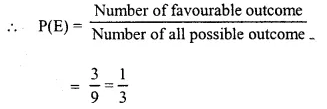(iii) An even number and a multiple of 3 are 6
which is one in number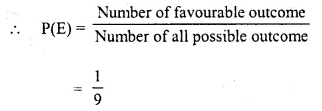(iv) An even number or a multiple of 3 are 2, 3, 4, 6, 8, 9, 10
which are 7 in numbersQuestion 2.
Hundred identical cards are numbered from 1 to 100. The cards are well shuffled and then a card is drawn. Find the probability that the number on the card drawn is :
(i) a multiple of 5.
(ii) a multiple of 6.
(iii) between 40 and 60.
(iv) greater than 85.
(v) less than 48.
Solution:
Number of cards = 100
Marked with numbers from 1 to 100
∴ Number of possible outcome = 100
(i) A multiple of 5 are 5, 10, 15 95, 100
which are 20 in numbers.
∴ Number of favourable outcome = 20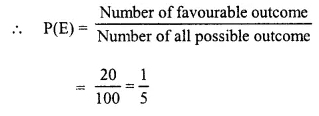(ii) A multiple of 6 are 6, 12, 18, 24, 90, 96
which are 16 in numbers.
∴ Number of favourable outcome =16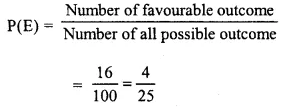(iii) Between 40 and 60 are 41, 42, ……. , 58, 59,
which are 19(iv) Greater than 85 are 86 to 100 which are 15 in numbers.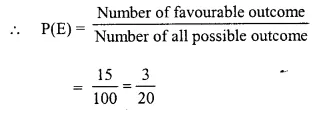(v) Less than 48 are 1 to 47 which are 47 in numbersQuestion 3.
From 25 identical cards, numbered, 1, 2, 3, 4, 5 ,…………, 24, 25 ; one card is drawn at random.
Find the probability that the number on the card drawn is a multiple of :
(i) 3
(ii) 5
(iii) 3 and 5
(iv) 3 or 5

Solution:
Number of identical cards = 25
Numbers marked on their are 1 to 25 i.e.
1, 2, 3, 4, 5, …. 21, 22, 23, 24, 25
∴ Number of possible outcome = 25
(i) Multiple of 3 are 3, 6, 9, 12, 15, 18, 21, 24
Which are 8 in numbers.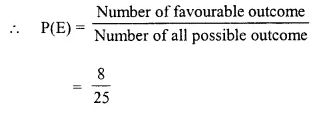(ii) Multiple of 5 are 5, 10, 15, 20, 25
which are 5 in numbers(iii) Multiple of 3 and 5 are = 15
which is 1 in number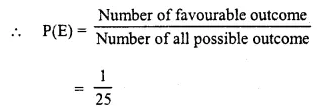(iv) Multiple of 3 or 5 are 3, 5, 6, 9, 10, 12, 15, 18, 20, 21, 24, 25
which are 12 in numbersQuestion 4.
A die is thrown once. Find the probability of getting a number :
(i) less than 3.
(ii) greater than or equal to 4.
(iii) less than 8
(iv) greater than 6.
Solution:
A die has 6 numbers i.e., 1, 2, 3, 4, 5, 6
∴ Number of possible outcome = 6
(i) Less than 3 are 1, 2 which are 2 in numbers(ii) Greater than or equal to 4 are 4, 5, 6
which are 3 in number.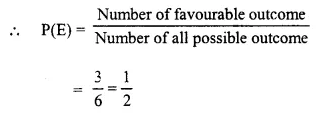(iii) Less than 8 are 1, 2, 3, 4, 5, 6
which are 6 in numbers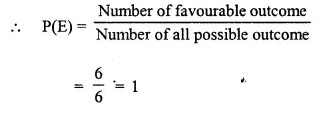(iv) Greater than 6 is nothing on the die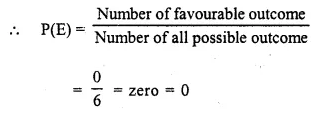Question 5.
A book contains 85 pages. A page is chosen at random. What is the probability that the sum of the digits on the page is 8 ?
Solution:
Number of pages of the book = 85
which are from 1 to 85
Number of possible outcome = 85
∴ Number of pages whose sum of its page is 8 are : 17, 26, 35, 44, 53, 62, 71, 80 and 8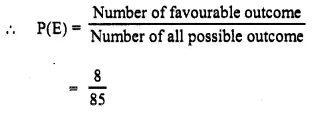Question 6.
A pair of dice is thrown. Find the probability of getting a sum of 10 or more if 5 appears on the first die.
Solution:
Numbers marked on each die = 6
∴ Total number of cases = 6 x 6 = 36
∵ Favourable come are (5, 5), (5, 6) as 5 appears on the first, which are 2 in number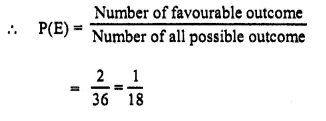Question 7.
If two coins are tossed once, what is the probability of getting :
(iii) both heads or both tails.
Solution:
∵ A coins has two faces Head and Jail or H, T
∴ Two coins are tossed
∴ Number of coins = 2 x 2 = 4
which are HH, HT, TH, TT
(i) When both are Head, then
∴ Number of outcome = 1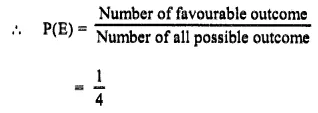(ii) At least one head, then
Number of outcomes = 3(iii) When both head or both tails, then 1
Number of outcomes = 2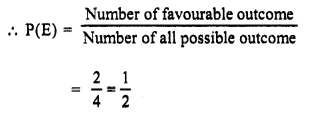Question 8.
Two dice are rolled together. Find the probability of getting :
(i) a total of at least 10.
(ii) a multiple of 2 on one die and an odd number on the other die.
Solution:
∵ A die has 6 faces which are 1, 2, 3, 4, 5,6
∴ On rolling two dice at a time, number of comes = 6 x 6 = 36
∴ Number of possible outcome = 36
(i) a total of atleast 10, the favourable can be (4,6), (5, 5), (5, 6), (6, 4), (6, 5), (6, 6) which are 6 in number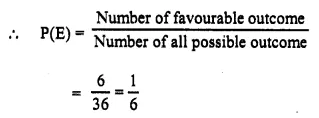(ii) A multiple of 2 on one die and an odd number on the other
∴ Outcome can be (2, 1), (2, 3), (g, 5), (4, 1), (4,3) , (4, 5), (6, 1), (6, 3), (6, 5), (1, 2), (3, 2), (5, 2), (1, 4), (3, 4), (5, 4), (1, 6), (3, 6), 5, 6) which are 18 in numbers.
Number of favourable outcomeQuestion 9.
A card is drawn from a well-shuffled pack of 52 cards. Find the probability that the card drawn is :
(ii) a red card.
(iii) a face card.
(iv) 5 of heart or diamond.
(v) Jack or queen.
(vi) ace and king.
(vii) a red and a king.
(viii) a red or a king.
Solution:
A pack of playing card has 52 cards
∴ Number of possible outcome = 52
∵ there are 13 cards of spade
∴ Number of favourable outcome = 13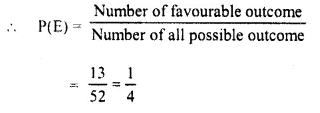(ii) A red card.
∵ There are 13 + 13 = 26 red card(iii) A face card.
∵ There are 3 x 4 = 12 faces which are red.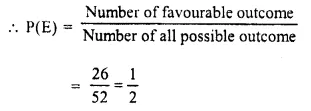(iv) 5 of heart or diamond.
∴ Number of cards =1 + 1=2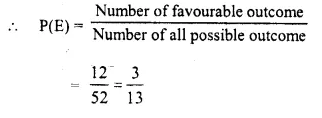(v) Jack or queen
There are 4 + 4 = 8 such cards(vi) ace and king.
There is no such card which is ace and king both
∴ P(E) = 0.
(vii) a red and a king
There are 2 such cards which are red kings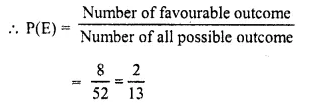(viii) a red or a king
There are 26 cards which are red in which 2 kings are red and 2 more kings which are black = 26 + 2 = 28Question 10.
A bag contains 16 coloured balls. Six are green, 7 are red and 3 are white. A ball is chosen, without looking into the bag. Find the probability that the ball chosen is :
(i) red
(ii) not red
(iii) white
(iv) not white
(v) green or red
(vi) white or green
(vii) green or red or white.
Solution:
Number of balls in a bag = 16
Green balls = 6
White balls = 3
Red balls = 7
∴ Total possible outcome =16
(i) Red balls = 7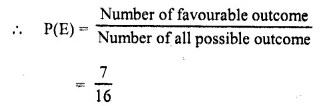(ii) Not red balls = 16-7 = 9(iii) White balls = 3(iv) Not white balls = 16 – 3= 13(v) Green or red balls = 6 + 7 = 13(vi) White or green balls = 3 + 6 = 9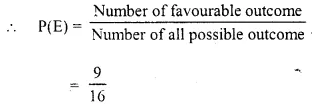(vii) Green or red or white balls =16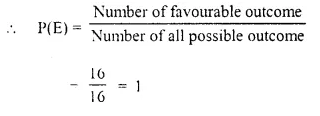Question 11.
A ball is drawn at random from a box .ontammg 12 white. 16 red and 20 green balls. Determine the probability that the ball drawn is:
(i) white
(ii) red
(iii) not green
(iv) red or white.
Solution:
Number of balls in a box
White = 12
Red = 16
Green = 20
Total balls = 12 + 16 +20 = 48
∴ Total possible outcome = 48
(i) White balls = 12
∴ Number of favourable outcome =12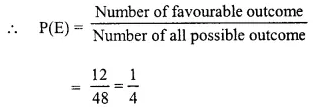(ii) Red balls = 16
∴ Number of favourable outcome =16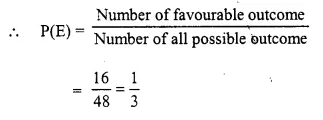(iii) Not green
Number of balls =12 + 16 = 28
∴ Number of favourable outcome = 28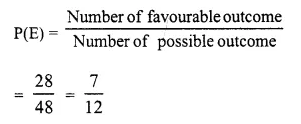(iv) Red or white balls =12 + 16 = 28
∴ Number of favourable outcome = 28Question 12.
A card is drawn from a pack of 52 cards. Find the probability that the card drawn is :
(i) a red card
(ii) a black card
(iv) an ace
(v) a black ace
(vi) ace of diamonds
(vii) not a club
(viii) a queen or a jack
Solution:
Number of cards in playing card deck = 52
∴ Number of possible outcome = 52
(i) A red card
There are 13 + 13 = 26 red cards in the deck
∴ Number of favourable outcome = 26(ii) A black card
There are 13 + 13 = 26 black cards
∴ Number of favourable outcome = 26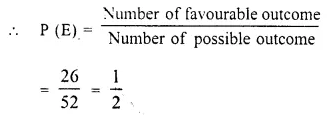There are 13 spade cards in the deck
∴ Number of favourable outcome = 13(iv) An Ace
There use 4 aces in the deck
Number of favourable outcome = 4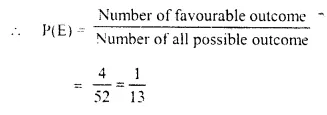(v) A black ace
There are two black aces in a deck
∴ Number of favourable outcome = 2
Number of cards in playing card deck = 52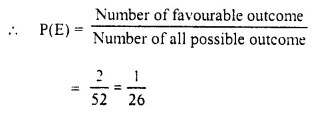(vi) Ace of diamonds
∴ There are only one ace of diamonds
∴ Number of favourable of outcome = 1(vii) Not a club
There are 13 x 3 = 39 cards which are not a club
∴ Number of favourable outcome = 39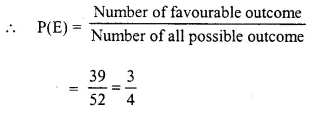(viii) A queen or a Jack
There are 4 queen cards and 4 Jack cards
∴ Number of favourable outcome = 4 + 4 = 8Question 13.
Thirty identical cards are marked with numbers 1 to 30. If one card is drawn at random, find the probability that it is :
(i) a multiple of 4 or 6.
(ii) a multiple of 3 and 5
(iii) a multiple of 3 or 5
Solution:
There are 30 cards which are marks with numbers 1 to 30 and one card is drawn
(i) A multiple of 4 or 6.
∴ There are multiple of 4 or 6 = 4,6, 8, 12, 16, 18, 20, 24, 28, 30 which are 10
∴ Number of favourable outcome = 10(ii) A multiple of 3 and 5 are 15, 30 which are 2
∴ Number of favourable outcome = 2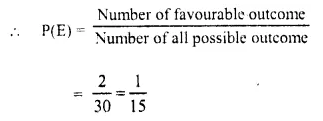(iii) A multiple of 3 or 5
which are 3, 5, 6, 9, 10, 12, 15, 18, 20, 21, 24, 25, 27, 30 which are 14
∴ Number of favourable outcome = 14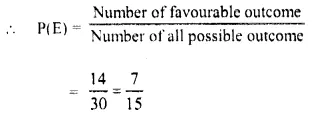Question 14.
In a single throw of two dice, find the probability of :
(i) a doublet
(ii) a number less than 3 on each dice.
(iii) an odd number as a sum.
(iv) a total of at most 10.
(v) an odd number on one dice and a number less than or equal to 4 on the other dice.
Solution:
Number of dice thrown = 2
Each die has 1 to 6 numbers on its faces Number of possible outcomes = 6 X 6 = 36
(i) A doublet : These can be (1, 1), (2, 2),(3, 3), (4, 4), (5, 5) and (6, 6) which are 6
∴ Number of favourable outcome = 6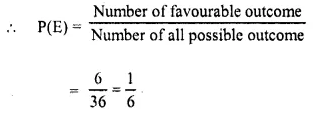(ii) A number less than 3 on each die which can be (1, 1), (1, 2), (2, 1), (2, 2) which are 4 in numbers
∴ Number of favourable outcome = 4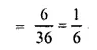(iii) An odd number as a sum which can be (1, 1), (1,3), (1,5), (2, 1), (2,3), (2,5), (3, 1), (3,3), (3,5), (4, 1), (4,3), (4, 5), (5,1),(5,3), (5,5), (6, 1), (6, 3), (6, 5) which are 18 in numbers.
∴ Number of favourable outcome = 18(iv) Total of at most 10
Which can be = 36 – 3 (which can be (5, 6), (6, 6), (6, 5) = 33
∴ Number of favourable outcome = 33(v) An odd number on one die and a number less than or equal to 4 on the other die
(1, 1), (1,2), (1,3), (1,4), (3,1), (3,2), (3, 3), (3,4) , (5, 1), (5, 2), (5, 3), (5, 4), (2, 1), (3, 1), (4, 1),(1, 3), (2, 3), (2, 5), (3, 1), (3, 5), (4, 1), (4, 3), (4,5) which are 23 in numbers
∴ Number of favourable outcome = 23Hope given Selina Concise Mathematics Class 10 ICSE Solutions Chapter 25 Probability Ex 25B  are helpful to complete your math homework.

If you have any doubts, please comment below. Learn Insta try to provide online math tutoring for you.